##1. Elation definition

These are projectivities characterized by the existence of a line (a) consisting entirely of fixed points, no other fixed point existing outside this line, and a special point A on this line such that all lines through A remain invariant under the map. Line (a) is called the axis and point A the center of the elation.
It can be easily shown that the requirement, to have a point A such that all lines through it remain invariant is a redundant one. It is implied by the property of the axis to include all the fixed points of the map and not having other fixed points outside.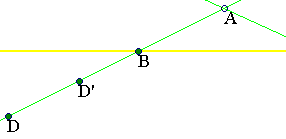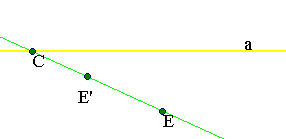In fact, consider two points {D,E} and their images {D'=F(D), E'=F(E)} under the elation. Lines {DD',EE'} are invariant under F and must intersect at a point A on the axis (a) of the elation. If this were not the case and A were outside (a), then A, being the intersection point of two invariant lines would be fixed and outside (a) contradicting the assumption on F.

##2. Harmonic homologies composition

 The product FC = FBFA (composition of transformations) of two harmonic perspectivities FA, FB with the same axis (e) and different centers A, B is an elation (FC) with axis (e) and center the intersection point C of (e) and the line AB.
 The product F' = FAFB of the harmonic perspectivities of the previous perspectivities FA, FB in reverse order is the inverse of FC. It is also an elation with the same center C and axis (e) but different from FC. For every point X, the images Z=FC(X), Z*=F'(X) are harmonic conjugate with respect to {X,C}.
 The map Z --> Z* is the commutator F'(FC)-1 = (FAFB)2 = F'2, which is also an elation with the same center and axis as FC.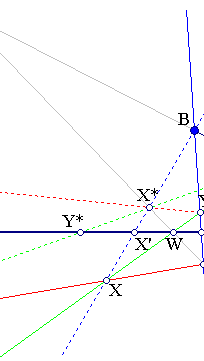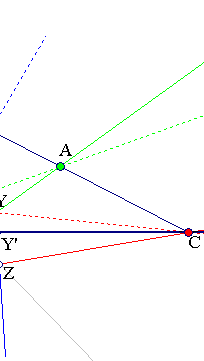Take Y=FA(X), Z=FB(Y) for an arbitrary point X. Points {X,Z,C} are collinear since (X,Y,A,X')=(Y,Z,B,Y')=-1. This proves . Point X*=FB(X) is on line CY because of the harmonic relation. Line X*Z intersects (e) at W, which is the intersection of lines {AX, e} because of the harmonic bundle at C. For the same reason (X*,Z,W,W')=-1, by projecting from X => (B,C,A,W')=-1 and by projecting this from X* => (C,X,Z,Z*)=-1.  follows from the preceding.

##3. Elation composition

 The product G of an elation FE, with center E, and a harmonic perspectivity FC, with center C, the two maps sharing the same axis (a), is a harmonic perspectivity FD. The center D of FD lying on line EC.
 Every elation FE is the product of two harmonic perspectivities FC*FD, the two perspectivities sharing with FE the same axis. This decomposition can be done in infinite many ways by selecting a point C arbitrarily, outside the axis (a) of the elation.
 The product of three harmonic perspectivities sharing the same axis (a) and having their centers {A,B,C} aligned is a harmonic perspectivity.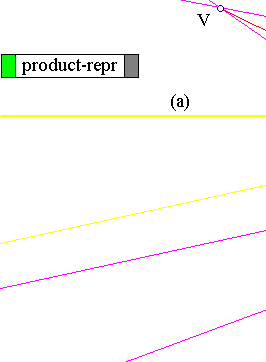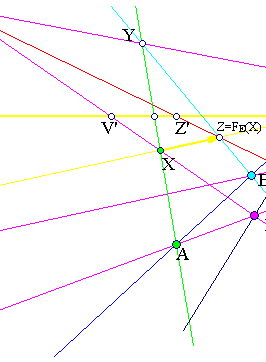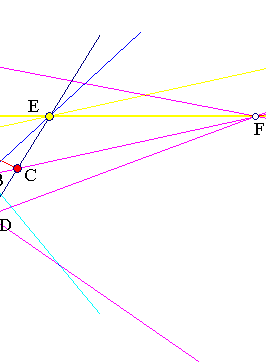Line CE is invariant under the composition G = FCFE. Point E is a fixed point of G. Hence G restricted on CE is represented by a Moebius transformation (ax)/(cx+d), taking x=0 to represent E. Then the other fixed point will satisfy cx+(d-a)=0, and since (d-a)2 is the determinant of the Moebius transformation in this case, there will be a second fixed point at x=(a-d)/c. Thus the composition G will fix the line (a) and the point D on CE corresponding to this value for x. Thus G will be a homology. It is then easily seen that the homology coefficient will be -1 by considering the cross ratio (C,Z',Z,V)=-1 , which by the definition of harmonic perspectivity at C, implies through radial projection from E that (V',D,X,V)=-1. Hence the map is a harmonic perspectivity. This proves . [2,3] are consequences of .

##4. Remarks

Remark-1 Previous figure shows the representation of the elation as a product of two harmonic perspectivities {FA, FB} with common axis. Y=FA(X), Z=FB(X), V = FC(X).
Remark-2 The figure below shows the application F(C) = D defined by a line (a) and two points {A,B} not on it. It is given by constructing first F as intersection of (a) and BC and then taking the intersection D of lines {EC, AF}. This map is identical with the elation with axis (a), center E and mapping B to A. This elation however is not one of {FBFA, FAFB}.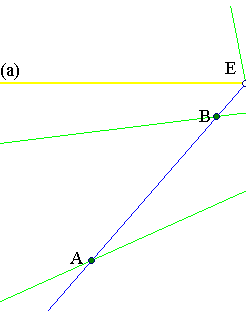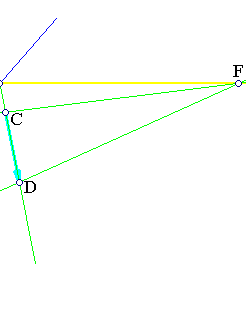Remark-3 An elation with axis (a) and center E, as above, cannot have a second fixed point E' different from E along AB. This would contradict its definition. Consequently the line-projectivity (f) induced on the invariant lines passing through E cannot be an involution. This would imply the existence of a second fixed point on line AB.
Remark-4 By selecting a projective basis in the line AB we can represent (f) of the previous remark through a Moebius transformation. This, according to remark-3 will have only one fixed point (E) on line BC, thus it is a parabolic Moebius transform.